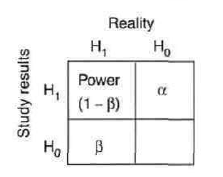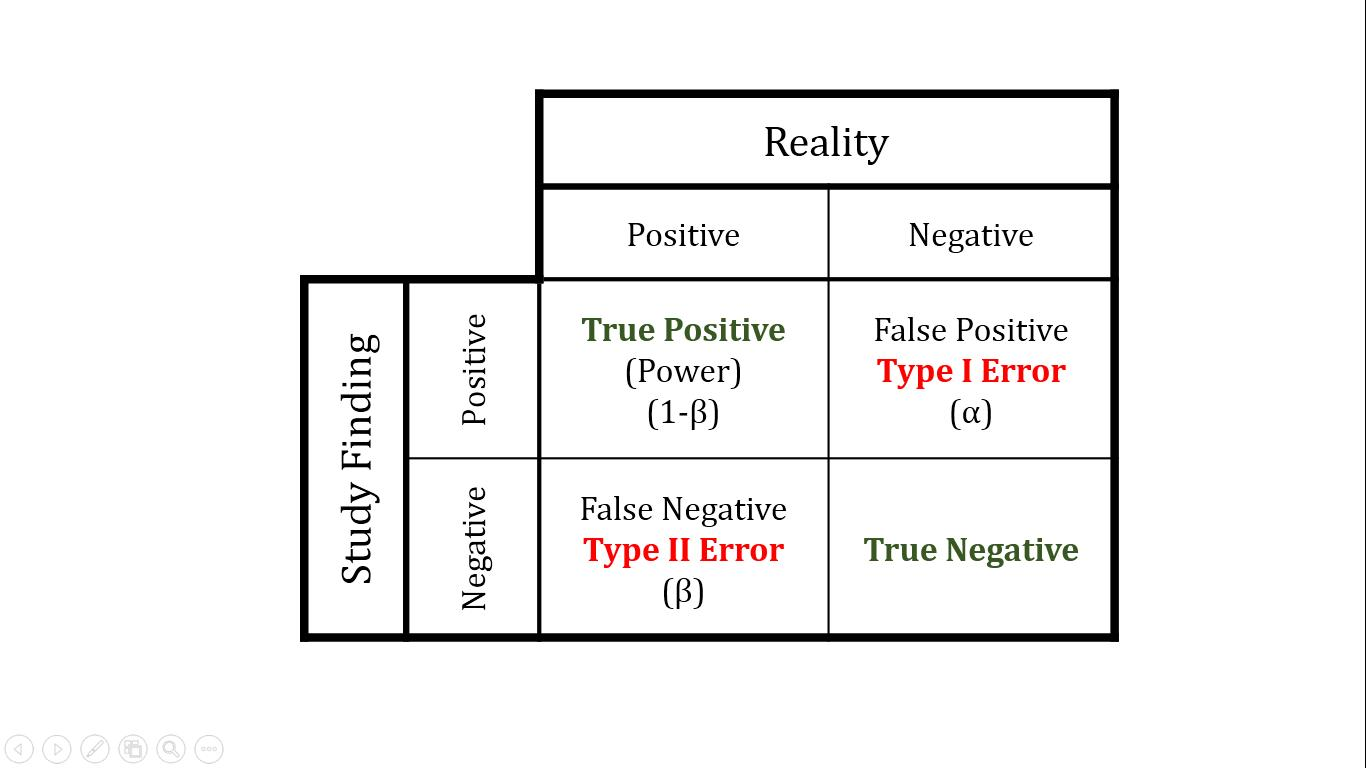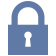Updated: 4/5/2022

# Statistical Hypotheses and Error

0%
Topic
Review Topic
0
0
N/A
N/A
Questions
6
0
0
0%
0%
Evidence
3
0
0
0%
0%
Videos / Pods
1
Topic
Images• Hypotheses
• hypothesis of no difference
• e.g., there is no link between disease and risk factor
• Alternative hypothesis (H1)
• hypothesis of difference
• e.g., there is a link between disease and risk factor
• Type I Error (False Positive)
• Stating there is an association when none exits
• incorrectly rejecting null hypothesis
• α = probability of type I error
• p = probability that results as or more extreme than those of the study would be observed if the null hypothesis were true
• general rule of thumb is that statistical significance is reached if p < 0.05
• Type II Error (False Negative)
• Stating there is no effect when an effect exists
• incorrectly accepting null hypothesis
• β = probability of type II error
• Power (True Positive)
• Probability of correctly rejecting null hypothesis
• power = 1 - β
• Power depends on
• sample size
• increasing sample size increases power
• size of expected effect
• increasing effect size increases power
• True Negative
• Probability of correctly accepting null hypothesis
• Confidence Interval
• Range of values associated with a confidence level indicating the likelihood that the true population value of a parameter falls within that range
• usually done with 95% confidence interval (2 standard deviations from the mean)
• e.g., based on our study data, we are 95% confident that the average salary of a teacher lies between \$30,000-45,000/year
• Confidence interval is calculated from statistics generated from the studied data
• Smaller confidence intervals suggest better precision of the data
• Larger confidence intervals suggest less precision of the data
• If confidence intervals of 2 groups overlap, there is no statistically significant difference
• A Priori Versus Post Hoc Analysis
• A priori comparisons
• comparisons planned prior to data analysis
• planning dependent on knowledge researchers have prior to conducting statistical tests
• Post hoc analysis
• researcher decides additional comparisons to make after viewing data
• choices dependent on knowledge researchers have gained after conducting statistical tests
• e.g., a test is run that says there is a difference between groups A, B, and C
• post hoc analysis would involve comparing group A to group B, B to C, and A to C to see between which groups the difference lies
• one potential hazard is an increased likelihood of spurious statistical associations

You have never rated this topic.

Thank you. You can rate this topic again in 12 months.

Flashcards (0)
Cards
1 of 0
Questions (6)Sorry, this question is forSorry, this question is for

(M1.ST.14.20) You are conducting a study comparing the efficacy of two different statin medications. Two groups are placed on different statin medications, statin A and statin B. Baseline LDL levels are drawn for each group and are subsequently measured every 3 months for 1 year. Average baseline LDL levels for each group were identical. The group receiving statin A exhibited an 11 mg/dL greater reduction in LDL in comparison to the statin B group. Your statistical analysis reports a p-value of 0.052. Which of the following best describes the meaning of this p-value?

QID: 104026

There is a 95% chance that the difference in reduction of LDL observed reflects a real difference between the two groups

19%

(17/89)

There is a 5% chance of observing a difference in reduction of LDL of 11 mg/dL or greater even if the two medications have identical effects

24%

(21/89)

Though A is more effective than B, there is a 5% chance the difference in reduction of LDL between the two groups is due to chance

37%

(33/89)

This is a statistically significant result

7%

(6/89)

If 100 permutations of this experiment were conducted, 5 of them would show similar results to those described above

2%

(2/89)

M 1 E

Select Answer to see Preferred Response

(M1.ST.14.77) A grant reviewer at the National Institutes of Health is determining which of two studies investigating the effects of gastric bypass surgery on fasting blood sugar to fund. Study A is spearheaded by a world renowned surgeon, is a multi-center study planning to enroll 50 patients at each of 5 different sites, and is single-blinded. Study B plans to enroll 300 patients from a single site and will be double-blinded by virtue of a sham surgery for the control group. The studies both plan to use a t-test, and they both report identical expected treatment effect sizes and variance. If the reviewer were interested only in which trial has the higher power, which proposal should he fund?

QID: 103894

Study A, because it has a superior surgeon

0%

(0/54)

Study A, because it is a multi-center trial

9%

(5/54)

Study B, because it has a larger sample size

46%

(25/54)

Study B, because it is double blinded

24%

(13/54)

Both studies have the same power

2%

(1/54)

M 1 E

Select Answer to see Preferred Response

(M1.ST.13.68) You submit a paper to a prestigious journal about the effects of coffee consumption on mesothelioma risk. The first reviewer lauds your clinical and scientific acumen, but expresses concern that your study does not have adequate statistical power. Statistical power refers to which of the following?

QID: 103885

The probability of detecting an association when no association exists.

15%

(8/55)

The probability of detecting an association when an association does exist.

58%

(32/55)

The probability of not detecting an association when an association does exist.

15%

(8/55)

The probability of not detecting an association when no association exists.

5%

(3/55)

The first derivative of work.

0%

(0/55)

M 1 E

Select Answer to see Preferred ResponseSorry, this question is for